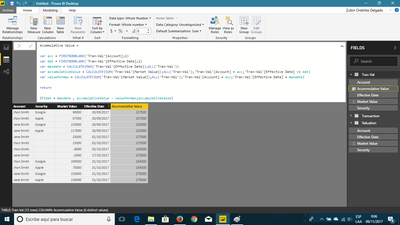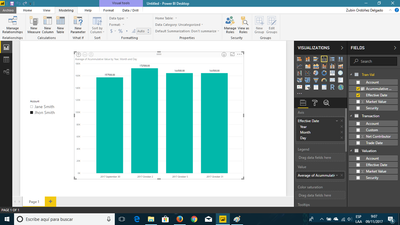cancel
Showing results for
Did you mean:

Fabric is Generally Available. Browse Fabric Presentations. Work towards your Fabric certification with the Cloud Skills Challenge.Frequent Visitor

## Combination chart with two separate tables, each having separate date field

I have two tables being brought in from MySQL called transaction and valuation.  Valuation shows the value of each security holding of each account holder for every month end date going back 5 years.  Transaction shows the net contribution for each contribution and withdrawal transaction for those same accounts but with staggered dates; i.e. they can be on any date, not just month end dates.  For example:My goal is to create a combination chart where transaction.TradeDate and valuation.EffectiveDate share the x-axis.  In the John Smith example, we would see Sep. 30, Oct. 2, Oct. 5 and Oct. 31 on the x-axis.

I would like bars of the combination chart to show the cumulative net contributions to the account between the beginning and ending valuation.EffectiveDate.  For Sep. 30, I would want the cumulative contributions to be \$157,500 (the total beginning market value of the account).  For Oct. 2, the amount would be \$172,500 (\$157,500 + \$15,000 contribution Oct. 2).  For Oct. 5 the amount would be \$164,500 (\$172,500 - \$8,000 withdrawal Oct. 5).  For Oct. 31, the amount would remain \$164,500.  I feel a PBI measure may be required here add in the total market value at September 30.

I would like the line of the combination chart to simply be the total market value of the account at each month end date.  This should be more simple: it would simply be \$157,500 at Sep. 30 and \$175,000 at Oct. 31 (the total market value of securities for John Smith).

Any help with this would be greatly appreciated.  Please advise if the above is unclear.

2 ACCEPTED SOLUTIONSSuper User

First of all you need to create a Date table and create a relationship between the two tables you already have, then assuming that you want to have this by year you need to create the following measures:

```Cumulative Net =
VAR datedim =
MAX ( 'Calendar'[Date] )
RETURN
TOTALYTD ( SUM ( Valuation[Market Value] ), 'Calendar'[Date] )
+ TOTALYTD ( SUM ( 'Transaction'[Net Contribution] ), 'Calendar'[Date] )```
```Filter_Non_Used_Dates =
IF (
SUM ( 'Transaction'[Net Contribution] ) + SUM ( Valuation[Market Value] )
= 0,
BLANK (),
1
)```

The just add the first measure to a column bar with the Calendar date in x-axis. second measure (Filter_Non_Used_Dates) is to filter out if you don't wnat to show on your chart the dates that have no transactions.Regards,

MFelix

Regards

Miguel Félix

Proud to be a Super User!

Check out my blog: Power BI em PortuguêsSolution Specialist

As an alternative...

1. Create a calculated table as a union of both tables.

`Tran-Val = UNION(    SELECTCOLUMNS    (        Valuation;"Account";Valuation[Account];"Security";Valuation[Security];"Market Value";Valuation[Market Value];    "Effective Date";Valuation[Effective Date]    );    SELECTCOLUMNS    (        'Transaction';"Account";'Transaction'[Account];"Security";'Transaction'[Custom];"Market Value";'Transaction'[Net Contributor];"Effective Date";'Transaction'[Trade Date]    ))`

NOTE: I created a new blank column in "Transaction" table to get the union correctly. (You can use power query)

2. Create a new calculated column inside Tran-Val table.

```Acummulative Value =

var acc = FIRSTNONBLANK('Tran-Val'[Account];1)
var dat = FIRSTNONBLANK('Tran-Val'[Effective Date];1)
var maxdate = CALCULATE(MAX('Tran-Val'[Effective Date]);ALL('Tran-Val'))
var accumulativeValue = CALCULATE(SUM('Tran-Val'[Market Value]);ALL('Tran-Val');'Tran-Val'[Account] = acc;'Tran-Val'[Effective Date] <= dat)
var valueformax = CALCULATE(SUM('Tran-Val'[Market Value]);ALL('Tran-Val');'Tran-Val'[Account] = acc;'Tran-Val'[Effective Date] = maxdate)

return

IF(dat = maxdate ; accumulativeValue - valueformax;accumulativeValue)```3.Then the final result is like this...I hope this helps

Regards

BILASolution

2 REPLIES 2Solution Specialist

As an alternative...

1. Create a calculated table as a union of both tables.

`Tran-Val = UNION(    SELECTCOLUMNS    (        Valuation;"Account";Valuation[Account];"Security";Valuation[Security];"Market Value";Valuation[Market Value];    "Effective Date";Valuation[Effective Date]    );    SELECTCOLUMNS    (        'Transaction';"Account";'Transaction'[Account];"Security";'Transaction'[Custom];"Market Value";'Transaction'[Net Contributor];"Effective Date";'Transaction'[Trade Date]    ))`

NOTE: I created a new blank column in "Transaction" table to get the union correctly. (You can use power query)

2. Create a new calculated column inside Tran-Val table.

```Acummulative Value =

var acc = FIRSTNONBLANK('Tran-Val'[Account];1)
var dat = FIRSTNONBLANK('Tran-Val'[Effective Date];1)
var maxdate = CALCULATE(MAX('Tran-Val'[Effective Date]);ALL('Tran-Val'))
var accumulativeValue = CALCULATE(SUM('Tran-Val'[Market Value]);ALL('Tran-Val');'Tran-Val'[Account] = acc;'Tran-Val'[Effective Date] <= dat)
var valueformax = CALCULATE(SUM('Tran-Val'[Market Value]);ALL('Tran-Val');'Tran-Val'[Account] = acc;'Tran-Val'[Effective Date] = maxdate)

return

IF(dat = maxdate ; accumulativeValue - valueformax;accumulativeValue)```3.Then the final result is like this...I hope this helps

Regards

BILASolutionSuper User

First of all you need to create a Date table and create a relationship between the two tables you already have, then assuming that you want to have this by year you need to create the following measures:

```Cumulative Net =
VAR datedim =
MAX ( 'Calendar'[Date] )
RETURN
TOTALYTD ( SUM ( Valuation[Market Value] ), 'Calendar'[Date] )
+ TOTALYTD ( SUM ( 'Transaction'[Net Contribution] ), 'Calendar'[Date] )```
```Filter_Non_Used_Dates =
IF (
SUM ( 'Transaction'[Net Contribution] ) + SUM ( Valuation[Market Value] )
= 0,
BLANK (),
1
)```

The just add the first measure to a column bar with the Calendar date in x-axis. second measure (Filter_Non_Used_Dates) is to filter out if you don't wnat to show on your chart the dates that have no transactions.Regards,

MFelix

Regards

Miguel Félix

Proud to be a Super User!

Check out my blog: Power BI em PortuguêsAnnouncements#### Power BI Monthly Update - November 2023

Check out the November 2023 Power BI update to learn about new features.#### Fabric Community News unified experience

Read the latest Fabric Community announcements, including updates on Power BI, Synapse, Data Factory and Data Activator.#### The largest Power BI and Fabric virtual conference

130+ sessions, 130+ speakers, Product managers, MVPs, and experts. All about Power BI and Fabric. Attend online or watch the recordings.Top Solution Authors
Top Kudoed Authors
Users online (3,835)